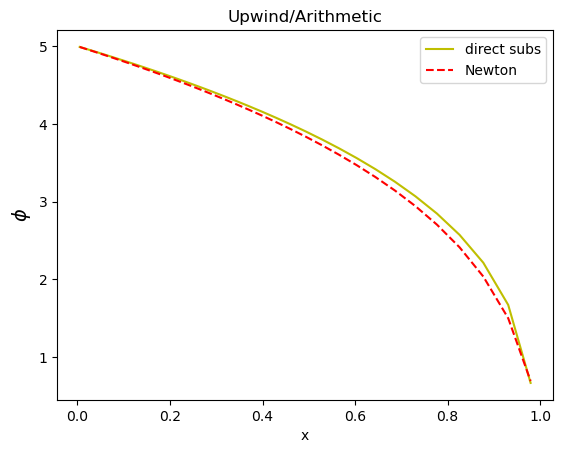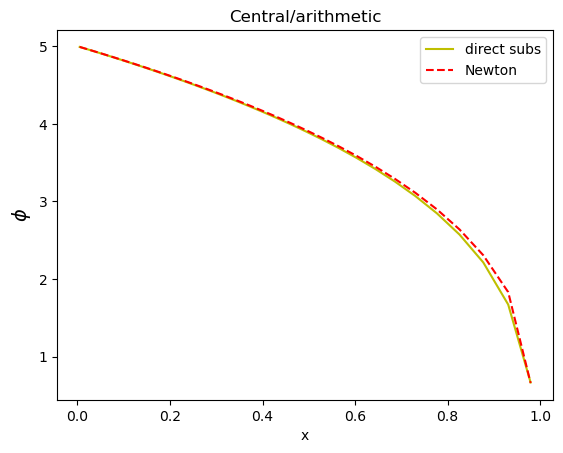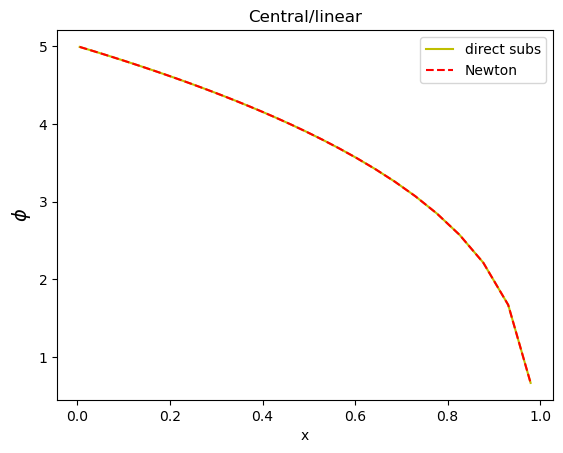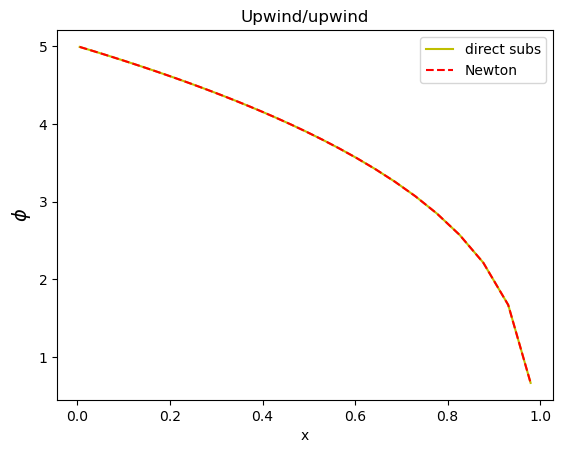# Choosing the right cell averaging method for linearized PDEs

## Choosing the right averaging method¶

In the previous post, I discussed a linearization procedure that could be used to solve a nonlinear diffusion equation. We saw that the linearization converted the nonlinear diffusion equation to a PDE with linear diffusion and convection terms. Here, I'm going to solve that equation again, but this time on a nonuniform mesh. My objective is to see what happens if we choose an averaging term that is not consistent with the chosen method for the discretization of the convection term.

### Another look at the equations¶

The equation that we solved was $$\frac{\partial\phi}{\partial t}+\nabla . (-D(\phi)\nabla \phi) =0$$ where we can linearize the diffusion term as $$D\left(\phi\right)\nabla \phi=D\left(\phi_{0}\right)\nabla \phi+\nabla \phi_{0}\left(\frac{\partial D}{\partial \phi}\right)_{\phi_{0}}\left(\phi-\phi_{0}\right).$$ Let's put it back in the original diffusion equation: $$\frac{\partial\phi}{\partial t}+\nabla . \left(-D\left(\phi_{0}\right)\nabla \phi-\nabla \phi_{0}\left(\frac{\partial D}{\partial \phi}\right)_{\phi_{0}}\left(\phi-\phi_{0}\right)\right) =0$$ Of course this is an approximation. The idea is that we gues a $\phi_0$, then solve the equation for $\phi$, and replace it in $\phi_0$ until the absolute or relative difference between them is less than a chosen small value. I write the above equation in a form that is actually used in a finite volume scheme with all the averaging on the cell faces. It reads $$\frac{\partial\phi}{\partial t}+\nabla . \left(-D\left(\phi_{0}\right)\nabla \phi\right)+\nabla.\left(-\nabla \phi_{0}\left(\frac{\partial D}{\partial \phi}\right)_{\phi_{0,face}}\phi\right) =\nabla.\left(-\nabla \phi_{0}\left(\frac{\partial D}{\partial \phi}\right)_{\phi_{0,face}}\phi_{0,face}\right)$$ Here's the trick: on the left hand side, we have, from left to right, a transient term, a diffusion term, a convection term, and on the right hand side, a divergence term. We can use any type of discretization for the convective term, as long as the same averaging term to calculate the face value on the right hand side, that would be:

Discretization Averaging
convectionTerm linearMean
convectionUpwindTerm upwindMean
convectionTvdTerm tvdMean

In this example, I'm going to show what will happen if you use an averaging technique that is not consistent with your chosen discretization method for the convective term.

### Implementation¶

I'm repeating the nonlinear diffusion model of the previous post, this time on a nonuniform grid. Two functions are created: the direct substitution, which is assumed to give the right solution, and a linearized equation, with the possibility of using central difference or upwind convection discretization and linear, upwind, and arithmetic averaging methods.

### Definition of functions in JFVM¶

In :
using JFVM, PyPlot, JFVMvis


### Linearized PDE (Newton method)¶

In :
function diffusion_newton(conv_method, avg_method)
L= 1.0 # domain length
Nx= 100
dx_min=L/Nx
x=[0.0, dx_min]
while x[end]<L
push!(x, x[end]+1.05(x[end]-x[end-1]))
end
x[end]=L
m= createMesh1D(x) # create a nonuniform 1D mesh
D0= 1.0 # diffusion coefficient constant
# Define the diffusion coefficientand its derivative
D(phi)=D0*(1.0+phi.^2)
dD(phi)=2.0*D0*phi
# create boundary condition
BC = createBC(m)
BC.left.a .= 0.0
BC.left.b .= 1.0
BC.left.c .= 5.0
BC.right.a .= 0.0
BC.right.b .= 1.0
BC.right.c .= 0.0
Mbc, RHSbc = boundaryConditionTerm(BC)
# define the initial condition
phi0 = 0.0 # initial value
phi_old = createCellVariable(m, phi0, BC) # create initial cells
phi_val = copyCell(phi_old)
# initialize the solution for upwind case
phi_face = linearMean(phi_val)
# define the time steps
dt= 0.01*L*L/D0 # a proper time step for diffusion process
for i=1:10
err=100
Mt, RHSt = transientTerm(phi_old, dt)
while err>1e-10
# calculate the diffusion coefficient
Dface= harmonicMean(cellEval(D,phi_val))
# calculate the face value of phi_0
if avg_method==1
phi_face= linearMean(phi_val)
elseif avg_method==2
phi_face= arithmeticMean(phi_val)
elseif avg_method==3
phi_face= upwindMean(phi_val, u)
end
# calculate the velocity for convection term
# diffusion term
Mdif= diffusionTerm(Dface)
# convection term
if conv_method==1
Mconv= convectionTerm(u)
elseif conv_method==2
Mconv= convectionUpwindTerm(u)
end
# divergence term on the RHS
RHSlin= divergenceTerm(u*phi_face)
# matrix of coefficients
M= Mt-Mdif+Mconv+Mbc
# RHS vector
RHS= RHSbc+RHSt+RHSlin
# call the linear solver
phi_new= solveLinearPDE(m, M, RHS)
# calculate the error
err= maximum(abs.(phi_new.value .- phi_val.value))
# assign the new phi to the old phi
phi_val=copyCell(phi_new)
end
phi_old= copyCell(phi_val)
#visualizeCells(phi_val)
end
return phi_val
end

Out:
diffusion_newton (generic function with 1 method)

### Direct substitution¶

In :
function diffusion_direct()
L= 1.0 # domain length
Nx= 100
dx_min=L/Nx
x=[0.0, dx_min]
while x[end]<L
push!(x, x[end]+1.05(x[end]-x[end-1]))
end
x[end]=L
m= createMesh1D(x) # create a nonuniform 1D mesh
D0= 1.0 # diffusion coefficient constant
# Define the diffusion coefficientand its derivative
D(phi)=D0*(1.0+phi.^2)
dD(phi)=2.0*D0*phi
# create boundary condition
BC = createBC(m)
BC.left.a .= 0.0
BC.left.b .= 1.0
BC.left.c .= 5.0
BC.right.a .= 0.0
BC.right.b .= 1.0
BC.right.c .= 0.0
Mbc, RHSbc = boundaryConditionTerm(BC)
# define the initial condition
phi0 = 0.0 # initial value
phi_old = createCellVariable(m, phi0, BC) # create initial cells
phi_val = copyCell(phi_old)
# define the time steps
dt= 0.01*L*L/D0 # a proper time step for diffusion process
for i=1:10
err=100
Mt, RHSt = transientTerm(phi_old, dt)
while err>1e-10
# calculate the diffusion coefficient
Dface= harmonicMean(cellEval(D,phi_val))
# diffusion term
Mdif= diffusionTerm(Dface)
# matrix of coefficients
M= Mt-Mdif+Mbc
# RHS vector
RHS= RHSbc+RHSt
# call the linear solver
phi_new= solveLinearPDE(m, M, RHS)
# calculate the error
err= maximum(abs.(phi_new.value .- phi_val.value))
# assign the new phi to the old phi
phi_val=copyCell(phi_new)
end
phi_old= copyCell(phi_val)
#visualizeCells(phi_val)
end
return phi_val
end

Out:
diffusion_direct (generic function with 1 method)

### Case 1: upwind convection with arithmetic average¶

In :
phi_ds=diffusion_direct()
phi_n=diffusion_newton(2,2)
plot(phi_ds.domain.cellcenters.x, phi_ds.value[2:end-1], "y",
phi_n.domain.cellcenters.x, phi_n.value[2:end-1], "--r")
legend(["direct subs", "Newton"])
title("Upwind/Arithmetic")
xlabel("x")
ylabel(L"\phi", fontsize=14);As you can see, the converged solution of the Newton method, which uses inconsistent convection/averaging terms does not match the solution of the direct substitution solver.

### Case 2: central convection with arithmetic average¶

In :
phi_ds=diffusion_direct()
phi_n=diffusion_newton(1,2)
plot(phi_ds.domain.cellcenters.x, phi_ds.value[2:end-1], "y",
phi_n.domain.cellcenters.x, phi_n.value[2:end-1], "--r")
legend(["direct subs", "Newton"])
title("Central/arithmetic")
xlabel("x")
ylabel(L"\phi", fontsize=14);Again, choosing the inconsistent methods results in an erroneous solution (for the Newton method).

### Case 3: central convection with linear average¶

In :
phi_ds=diffusion_direct()
phi_n=diffusion_newton(1,1)
plot(phi_ds.domain.cellcenters.x, phi_ds.value[2:end-1], "y",
phi_n.domain.cellcenters.x, phi_n.value[2:end-1], "--r")
legend(["direct subs", "Newton"])
title("Central/linear")
xlabel("x")
ylabel(L"\phi", fontsize=14);Beautiful! The results match perfectly.

### Case 4: upwind convection with upwind average¶

In :
phi_ds=diffusion_direct()
phi_n=diffusion_newton(2,3)
plot(phi_ds.domain.cellcenters.x, phi_ds.value[2:end-1], "y",
phi_n.domain.cellcenters.x, phi_n.value[2:end-1], "--r")
legend(["direct subs", "Newton"])
title("Upwind/upwind")
xlabel("x")
ylabel(L"\phi", fontsize=14);Again, we get the correct result by choosing a right pair of discretization/averaging methods. Depending on the Peclet number (convective to diffusive rate ratio), using the upwind method gives a numerically more stable solution.
Updated: August 2021 to work with Julia 1.6